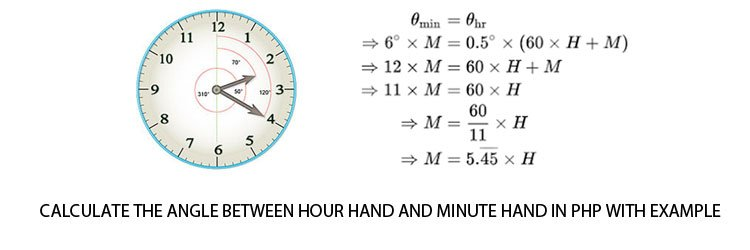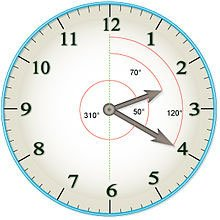## Calculate The Angle Between Hour Hand And Minute Hand In PHP [Example]

By Anand Lagad   December 7, 2017

Question:
12-hour analog clock and the time is given,

## calculate the angle between hour hand & minute hand in php.

Time: 02:20;The question is related to Clock Angle Problems.

Many times PHP Developers also facing such questions while interviewing.

To get hands-on experience in such questions you should study the Clock Angle Problem concept and formulae.

### Pin Points:

1. It is 12-hour analog clock so please start calculating angles and respective degree values from the mark of number 12 clockwise.
2. Please check the image for given time. Many of the interviewees get confused between the exact place of Hour Hand and Minute Hand. The time is 2:20, many of interviewees consider the Hour hand at 2. Hour Hand is slightly ahead of 2.
3. To calculate the angle between hour hand and minute hand we must
• Calculate the angle made by hour hand with respect to 12:00 in \$h hours and \$m minutes.
• Calculate the angle made by minute hand with respect to 12:00 in \$h hours and \$m minutes.
The difference between these two angles is the angle between two hands.
4. Draw a diagram as per above image for better understanding.

## calculate the angle between hour hand & minute hand in php.

``````<?php

// CALCULATE THE ANGLE BETWEEN HOUR HAND AND MINUTE HAND

// STEP 1. Set display errors on

ini_set('display_errors', 1);

// STEP 2. Initialise two variables for hour and minute as below,

\$h=2;  \$m=20;

\$angle=0;     // in degrees

// STEP 3. Define and call the method for angle calculation

function clculateHMAngle(\$h,\$m){

// validate the input

if (\$h < 0 || \$m < 0 || \$h >12 || \$m > 60)

{ echo "Please enter corrct input!"; die; }

if (\$h == 12)

\$h = 0;

if (\$m == 60)

\$m = 0;

//Calculate the angle moved by hour hand from the mark of number 12 clockwise

\$hour_angle = 0.5 * (\$h*60  +  \$m);  // 70 degrees

//Calculate the angles moved by minute hand from the mark of number 12 clockwise

\$minute_angle = 6 * \$m;  // 120 degrees

// Calculate the difference between hour hand angle and minute hand angle

\$angle = abs(\$hour_angle - \$minute_angle);   // 70 -120 = abs(-50) = 50 degrees

//Get the smallest angle between 360- \$angle and \$angle

\$angle = min( (360-\$angle), \$angle);  // 360-50, 50 = 50 Degrees

echo  \$angle;       // 50 Degrees

}

clculateHMAngle(\$h,\$m);

?>``````

### Explanation:

1. The Hour Hand moves 360 degrees in 12 hours i.e 720 minutes or 0.5 degrees in 1 minute. Therefore the formula for calculating hour hand angle is,
hour_angle = 0.5 * ( hours *60 + minutes);
Total time is converted into minutes and multiplied by 0.5 to get the number of degrees as a result.
2. The Minute hand moves 360 degrees in 60 minutes i.e 6 degrees in 1 minute. Therefore the formula for calculating minute hand angle is,
minute_angle = 6 * minutes;
The total number of degrees are stored in minute_angle as a result of minute hand angle.
3. By calculating the difference between these two angles we will get the angle between hour hand and minute hand.

Hope this helped! 🙂

Please comment if you have any doubt!